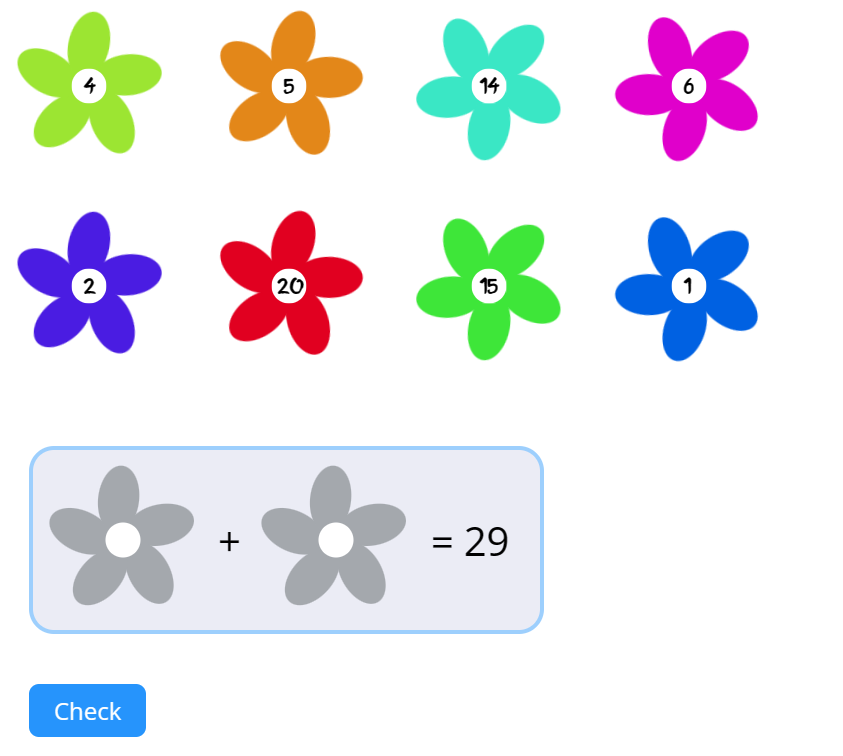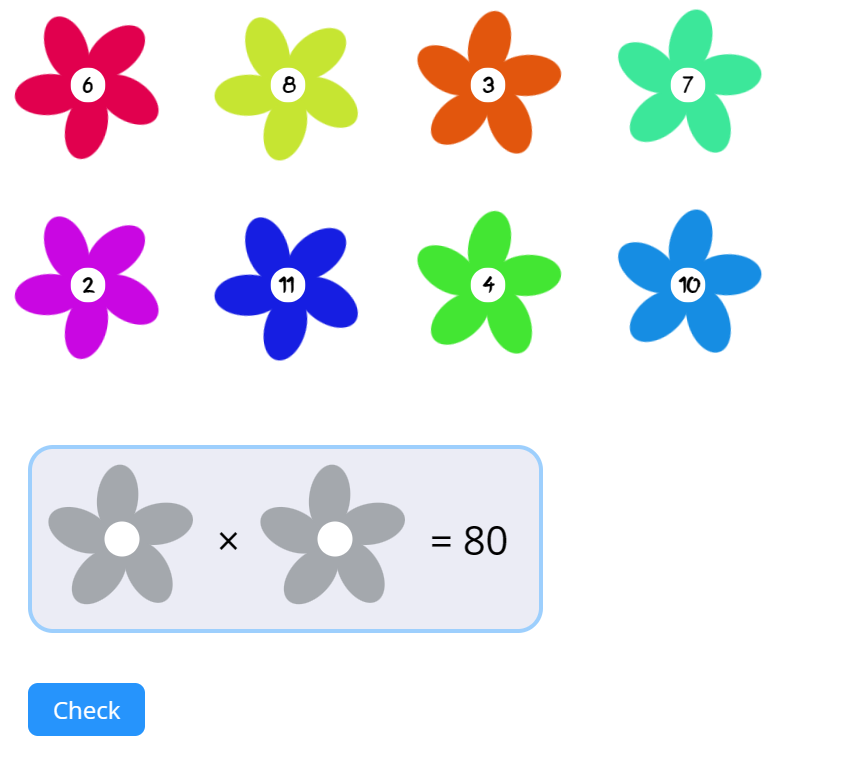MM Practice
×
Multiplication
Division
Place Value
Fractions & Decimals
Measurement
Statistics & Probability
Pre-Algebra
Money
Kindergarten
Geometry

# Make number sentences

#### Online practice for grades 1-4

You're given numbers (in flowers), and an answer to a math sentence. Drag two flowers to the empty slots so that the math sentence is true.

Choose any of the four operations — addition, subtraction, multiplication, or division.

You can also decide the number range of the numbers used, with the exception of division. This means your addition, subtraction, and multiplication sentences can be with one, two or three-digit numbers, as you wish. Or with negative numbers! For division, the numbers used come from the basic multiplication tables, up to 12 × 12 = 144. (For example, the multiplication fact 6 × 7 = 42 would give rise to the division fact 42 ÷ 7 = 6.)

Note: If you are using a phone, the game works best if you turn your phone to landscape mode.

Type:

Screenshots from the game:23
23
23
23
23
23
23
23
+
=